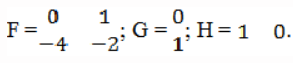# State Variable Analysis – Part II

State Variable Analysis – Part II

1. State variable analysis has several advantages overall transfer function as:

a) It is applicable for linear and non-linear and variant and time-invariant system
b) Analysis of MIMO system
c) It takes initial conditions of the system into account
d) All of the mentioned

Explanation: State variable analysis has several advantages overall transfer function as it is applicable for linear and non-linear and variant and time-invariant system, analysis of MIMO system, it takes initial conditions of the system into account.

2. The minimum number of states require to describe the two degree differential equation:

a) 1
b) 2
c) 3
d) 4

Explanation: The number of states required to describe a network is equal to the number of energy storing elements in the electrical network.

3. For a system with the transfer function H(s) = 3(s-2)/s3+4s2-2s+1 , the matrix A in the state space form is equal to:Explanation: Taking the inverse Laplace of the equation and converting it into differential equation and solving the state space by considering various matrices.

4. The transfer function Y(s)/U(s) of a system described by the state equations dx/dt=-2x+2u and y(t) = 0.5x is:

a) 0.5/(s-2)
b) 1/(s-2)
c) 0.5/(s+2)
d) 1/(s+2)

Explanation: Taking Laplace transform of the equation X(s) = 2U(s)/(s+2)
Then Y(s)/U(s) =1/(s+2).

5. A linear time invariant single input single output system has the state space model given by dx/dt=Fx+Gu, y=Hz, whereHere, x is the state vector, u is the input, and y is the output. The damping ratio of the system is:

a) 0.25
b) 0.5
c) 1
d) 2

Explanation: T(s) = 1/(s2+2s+4)
G = 0.5.

6. Given the matrixthe Eigen value are___________

a) 1,2,3
b) 1
c) -1,-2,-3
d) 0

Explanation: Solving the characteristic equation|SI-A| =0.

7. A transfer function of control system does not have pole-zero cancellation. Which one of the following statements is true?

a) System is neither controllable nor observable
b) System is completely controllable and observable
c) System is observable but uncontrollable
d) System is controllable but unobservable

Explanation: If the input-output transfer function of a linear time-invariant system has pole-zero cancellation, the system will be neither controllable nor observable.

8. The analysis of multiple input multiple output is conveniently studied by;

a) State space analysis
b) Root locus approach
c) Characteristic equation approach
d) Nicholas chart

Explanation: State space analysis is different from the transfer function approach as this can be used for the analysis of multiple input and multiple output system.

9. The state equation in the phase canonical form can be obtained from the transfer function by:

b) Direct decomposition
c) Inverse decomposition
d) Parallel decomposition

Explanation: The state equation from transfer function by parallel decomposition in the phase canonical form.

10. A logarithmic spiral extending out of the singular point is__________

a) Stable
b) Unstable focus
c) Conditionally stable
d) Marginally stable